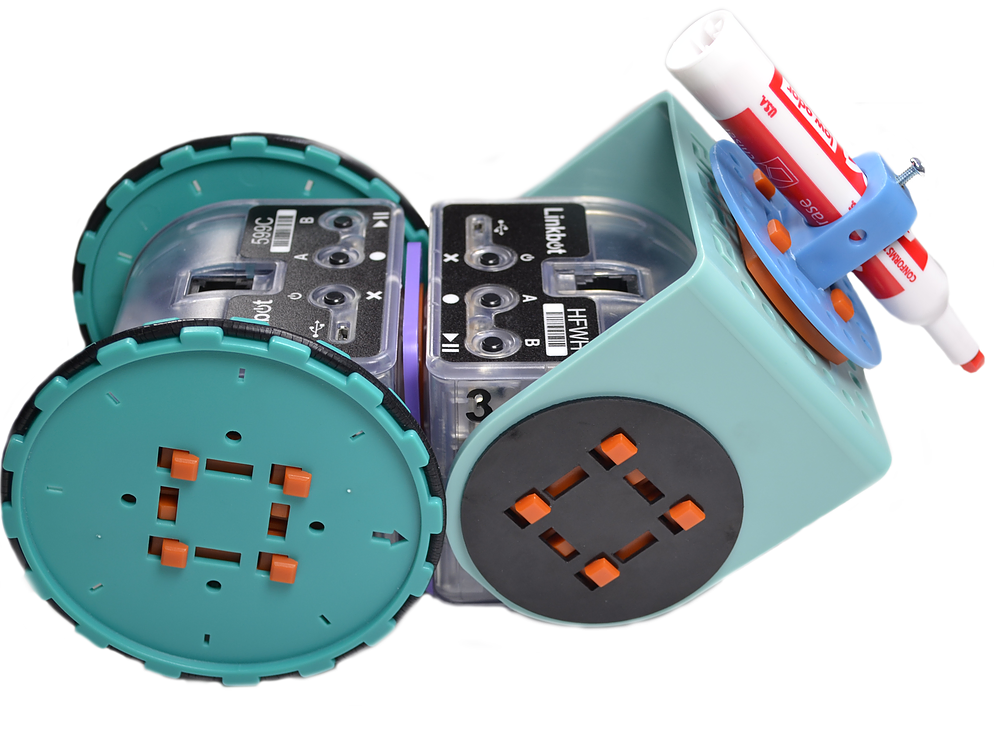Recent Posts

Archive

Tags

# [Project] Robotic drawing with the new Linkbot Sketch Pack!

The new Linkbot Sketch Pack adds fun drawing capabilities to Linkbot robots! Program the Linkbot to create all kinds of shapes and patterns as it moves over a paper or whiteboard surface, lowering the pen to draw and raising it to move to another area without drawing.The following program, written in Ch code, shows how to control the Linkbot so that it traces out a square. (PDF file with code available here.)

/* Square Drawing Demo

This demo shows how to program the robot to draw a square by using a pen with the Linkbot Sketch Pack accessories. Note that there are two adjustment factor variables (turn_adjfactor and line_adjfactor) that need to be specified, depending on the surface being used. (Trial and error required for best results.) */

// Initialize the robots (#1 for driving, #2 for raising/lowering pen) CLinkbotI robot1, robot2;

// Define variables int pen_angle = 30; // the pen angle through which the pen is raised and lowered int robot_to_pen_distance = 5; // the distance between the center of the driving robot and the pen position int side_length = 5; // the side length of the square to be drawn int count = 0; // counter for loop int turn_angle = 90; // the angle through which the robot will turn at each corner

double wheelradius = 2; // radius for large robot wheels used double trackwidth = 3.69; // distance between the two wheels (width of the robot's track) double turn_adjfactor = 1.1; // adjustment factor for turning at corners (explained later in comments) double line_adjfactor = 1.1; // adjustment factor for straight line moving (explained later in comments)

// The reason for the adjustment factors above is due to friction between the surface and the robot's wheels and // caster. The amount of friction will vary depending on the surface.

// The value of line_adjfactor is affected by the friction of the wheels and the caster together. // In other words, more friction means that the robot's moving distance needs to be increased to result in // moving the desired distance. For example, to move 5 inches, the robot may need to be told to move 5.3 inches. // Suggested range for this value is 1.05 to 1.2 (set in the "define variables" section above).

// The value of the turn_adjfactor is affected by the relationship between the wheels and caster. // If the friction of wheels is higher than that of the caster, then the value of turn_adjfactor // is less than 1.15. If not, the value of turn_adjfactor may be larger than 1.15. // Suggested range for this value is 1.1 to 1.35 (set in the "define variables" section above).

//***** main code *****//

// Raise the pen by specified angle before moving robot robot2.move(-pen_angle, NaN, pen_angle);

// Use loop to draw each side of the square while(count < 4) { // Move robot to put pen in proper position to start drawing robot1.driveDistance(robot_to_pen_distance_adj, wheelradius); // Pen down robot2.move(pen_angle, NaN, -pen_angle); robot2.delaySeconds(0.5); // Move robot to draw one of the square's sides robot1.driveDistance(side_length_adj,wheelradius); // Pen up robot2.move(-pen_angle, NaN, pen_angle); robot2.delaySeconds(0.5); // Back up a little bit at each corner to put pen in proper position for next side robot1.driveDistance(-robot_to_pen_distance_adj,wheelradius); // Turn the robot left robot1.turnLeft(turn_angle_adj, wheelradius, trackwidth);

count = count + 1; }

robot2.delaySeconds(0.5);

robot2.move(pen_angle, NaN, -pen_angle); //pen up